# Para Bellum

## Prepare for the worst riskRafael Ballestas

"Si vis pacem, para bellum", goes the old adage. If you want peace, prepare for war. In our case, the worst possible risky scenario our information assets could go into. While probability distributions, loss exceedance curves, simulated scenarios, etc, are all great for the quants in the office, at the end of the day big, important decisions need to be supported by single numbers that can be easily compared to one another. In risk management, this number is the Value at Risk or `VaR`. Fortunately, once you have one you have the other.

`VaR` measures this scenario by telling us beyond how much our losses will not go, with a certain degree of confidence, over a definite period of time. Thus a daily 1% `VaR` of \$10 million means that the probability that you will lose more than ten million is 1%, i.e., are 99% confident that the losses will not exceed that.

So we need to define over what time period our `VaR` will be taken and how extreme the worst-case scenario. Typical periods and confidences used in the industry are a single day or week, and confidence levels of 95% or 99%.

There are at least three workable ways to compute the value at risk:

1. Examining the distribution of the returns,

2. Using the loss exceedance curve (`LEC`)

The normal distribution is perhaps the most popular one for modeling real-word situations and natural phenomena, and with good reason. It could be used, to model the value of a portfolio over a one-year period, with mean return 10%, and standard deviation (volatility) 30%: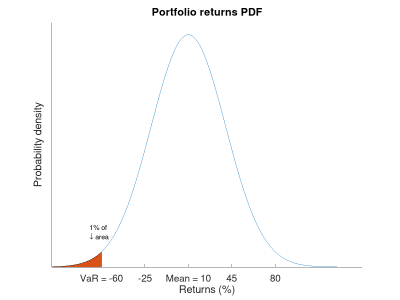Figure 1. Normal distribution of value

Knowing the probability distribution, which tells us probabilities of point values, we can find probabilities of ranges with the corresponding cumulative distribution function (`CDF`):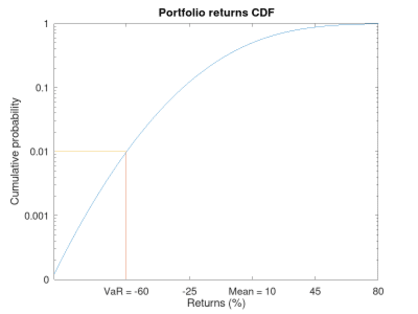Figure 2. Cumulative distribution function of value

Looks like a vertically reflected `LEC`. In a cumulative probability plot the `VaR` is just the x-value corresponding to the confidence.

We can use a spreadsheet, for this, with the `NORM.DIST` function. The probability that the loss exceeds 20% is

``````    =NORM.DIST(-20,10,30,1)
0.158655253931457
``````

i.e., around 15.8%. The 10 and 30 above are the distribution parameters, and the -20 is the value whose probability we need. Notice that it is negative, meaning a loss. The 1 means to make the computations cumulative.

We can also use the inverse function so that, given a probability, we get the point at which this probabilty is attained. It is the same process as above, but backwards.

At what point is the 1% probability? More exactly, for which value V is it true that the probability that the final value is less than or equal to V is 1%? That’s just the 1% `VaR`:

``````    =NORM.INV(0.01,10,30)
-59.7904362212252
``````

This is the 1% quantile, or the first percentile of the distribution, the point under which the remaining 1% of points are, weighing by the probability. Thus the Value at Risk in this example will be 59.8% of what we invested. Had we invested \$100 million, then we know the `VaR` is \$59.8 million, and hence that the losses will not exceed that amount in 99% of the cases, only in that rare 1%. Notice that the `VaR`, being a single figure, does not tell us exactly or otherwise what the losses might be in that catastrophic 1%. But if we are ready to lose that much, we are halfway prepared for the metaphoric war.

## Get started with Fluid Attacks' Vulnerability Management solution right now

The tail (or conditional) value at risk, or `TVaR` (`CVaR`) for short, tries to fill that void by giving us the expected value or mean in the catastrophe region, i.e., in case of a `VaR` breach. Much like the actual mean of a distribution is a center of gravity of sorts, where we could "hold" the `PDF` in balance, besides being the value with more repetitions if we repeatedly draw numbers from such a distribution: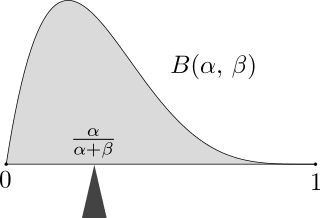Figure 3. Expected value of a beta distribution. Via Wikimedia.

The `TVaR` is thus the expected value of the loss, given that the `VaR` has been surpassed. In terms of the above analogy, it is the center of gravity of the "catastrophe" region of the distribution plot: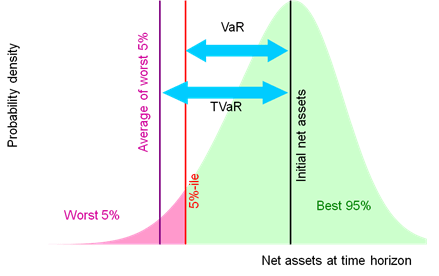Figure 4. Illustration of `VaR` and `TVaR`. Via Nematrian.

In our case, since we are mainly interested in cybersecurity risk, which we quantify via simulations, we can always re-run them and aggregate the results differently in order to obtain the density function and recreate the example above. But given that the main result of our simulations was a loss exceedance curve: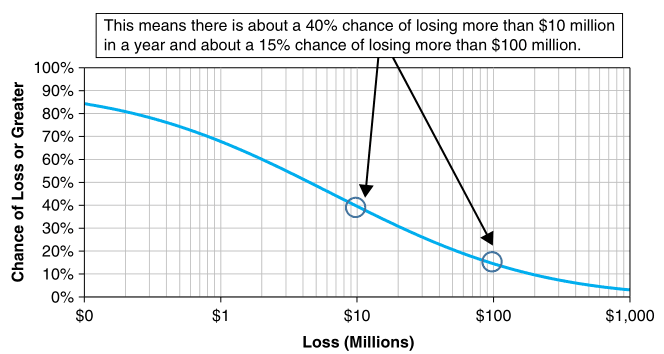Figure 5. Loss exceedance curve

We can just use this to obtain the `VaR`, just like we did with the distribution `CDF`. This graph is already cumulative, so there is no need to compute areas under the curve behind the scenes. We simply obtain the value in millions corresponding to the percentage of the scenario in which we are interested. In this particular graph, the 5% yearly `VaR` appears to be \$500 million (recall that this graph has a logarithmic scale in the x-axis). The 1% is not even visible here, but at least that tells us that it must be beyond \$1000 million.

Monitoring a short-termed `VaR` can be usefulto evaluate the performance of risk management or to understand events from the past: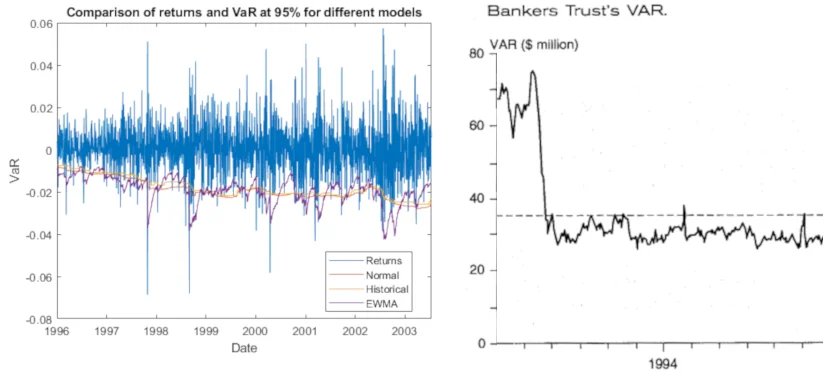Figure 6. Artificial VaR monitoring (via MathWorks) and real example from Bankers Trust, via .

In the first we see a steady, if slow, decline in `VaR` over the years. Also notice how the returns are almost always above their corresponding values-at-risk, save for a few rare breaches, which is to be expected.

In the image to the right there is an interesting moment around February 1994, where there is a sharp decrease in the `VaR`, after which it pretty much stays stable under the risk appetite line (dashed). This phenomenon is explained in Jorion’s book  as a response to a rise in interest rates at that moment, which was just as sharp as the decrease in the `VaR`.

However, a decreasing `VaR` is not all. Shying away from investments to keep the `VaR` low will, by symmetry, mean lower chance of great returns:

"A risk manager has two jobs: make people take more risk the 99% of the time it is safe to do so, and survive the other 1% of the time. `VaR` is the border."

— Aaron Brown

So, the VaR tells us in a single number what can happen with an investment or any risky situation the worst that might happen. However its greatest strength is also where it falls short. This particular number, while it gives an upper bound for the losses, is also unable to tell us anything else about what happens in that 1% of the cases. The `TVar` tries to fill this void, but it is still just a number, meaning that it inherits this same weakness.

## References

1. S. Benninga and Z. Wiener (1998). Value-at-Risk (`VaR`). Mathematica in Education and Research 7(4)

2. P. Jorion (2006). Value at Risk: The New Benchmark for Managing Financial Risk. McGraw-Hill.

3. N. Pearson (2002). Risk Budgeting: portfolio problem solving with value-at-risk. Wiley.

Share

Tags:

## Recommended blog posts

You might be interested in the following related posts.Definition, implementation, importance and alternativesKeep tabs on this proposal from the Biden-Harris AdminVulnerability scanning and pentesting for a safer webDefinitions, classifications and pros and consIs your security testing covering the right risks?How this process works and what benefits come with itGet an overview of vulnerability assessmentBenefits of continuous over point-in-time pentesting

## Start your 21-day free trial

Discover the benefits of our Continuous Hacking solution, which hundreds of organizations are already enjoying.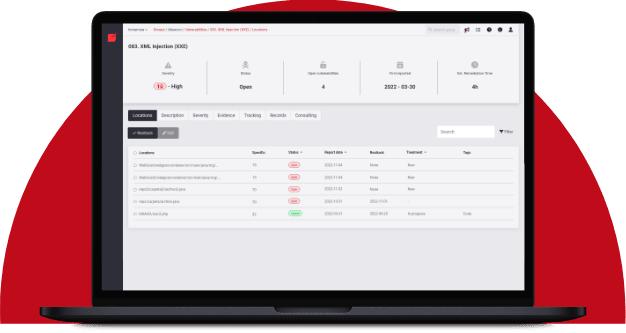Hacking software for over 20 years

Fluid Attacks tests applications and other systems, covering all software development stages. Our team assists clients in quickly identifying and managing vulnerabilities to reduce the risk of incidents and deploy secure technology.